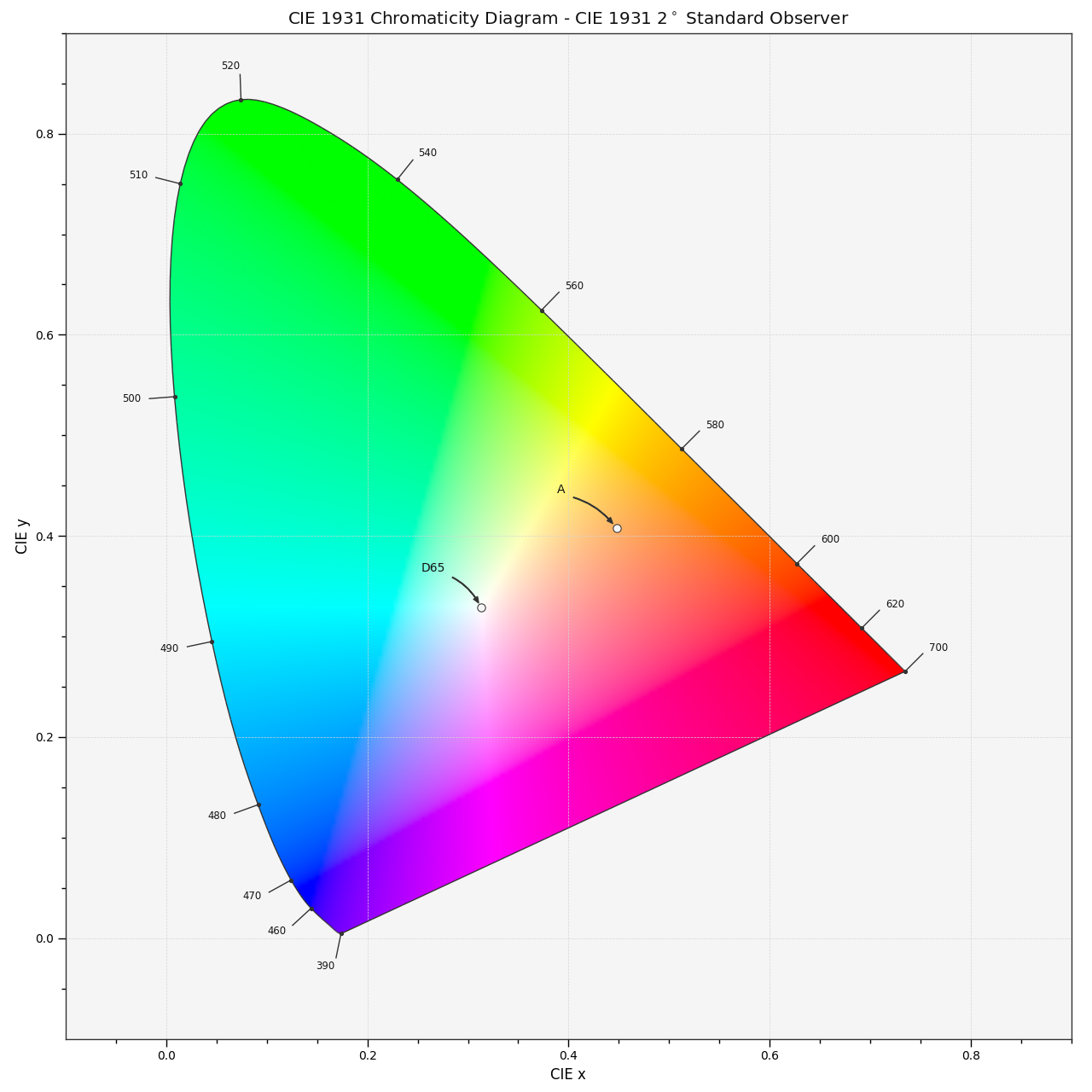# colour.plotting.diagrams.plot_sds_in_chromaticity_diagram¶

colour.plotting.diagrams.plot_sds_in_chromaticity_diagram(sds, cmfs='CIE 1931 2 Degree Standard Observer', annotate_parameters=None, chromaticity_diagram_callable=<function plot_chromaticity_diagram>, method='CIE 1931', **kwargs)[source]

Plots given spectral distribution chromaticity coordinates into the Chromaticity Diagram using given method.

Parameters
• sds (array_like or MultiSpectralDistributions) – Spectral distributions or multi-spectral distributions to plot. sds can be a single colour.MultiSpectralDistributions class instance, a list of colour.MultiSpectralDistributions class instances or a list of colour.SpectralDistribution class instances.

• cmfs (unicode, optional) – Standard observer colour matching functions used for Chromaticity Diagram bounds.

• annotate_parameters (dict or array_like, optional) – Parameters for the plt.annotate() definition, used to annotate the resulting chromaticity coordinates with their respective spectral distribution names if annotate is set to True. annotate_parameters can be either a single dictionary applied to all the arrows with same settings or a sequence of dictionaries with different settings for each spectral distribution.

• chromaticity_diagram_callable (callable, optional) – Callable responsible for drawing the Chromaticity Diagram.

• method (unicode, optional) – {‘CIE 1931’, ‘CIE 1960 UCS’, ‘CIE 1976 UCS’}, Chromaticity Diagram method.

Other Parameters

**kwargs (dict, optional) – {colour.plotting.artist(), colour.plotting.diagrams.plot_chromaticity_diagram(), colour.plotting.render()}, Please refer to the documentation of the previously listed definitions.

Returns

Current figure and axes.

Return type

tuple

Examples

>>> from colour import ILLUMINANTS_SDS
>>> A = ILLUMINANTS_SDS['A']
>>> D65 = ILLUMINANTS_SDS['D65']
>>> plot_sds_in_chromaticity_diagram([A, D65])
(<Figure size ... with 1 Axes>, <matplotlib.axes._subplots.AxesSubplot object at 0x...>)